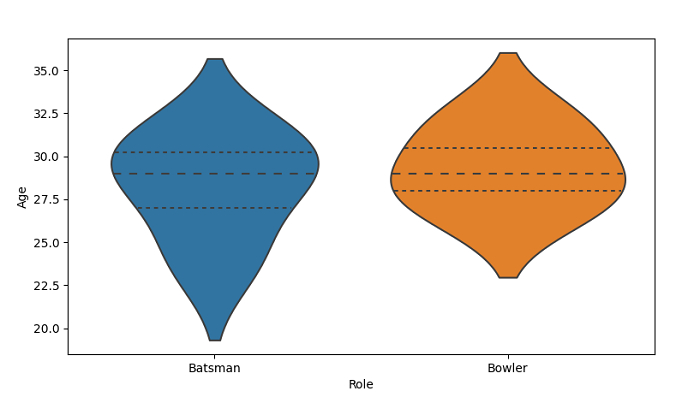# Python Pandas - Draw a violin plot and set quartiles as horizontal lines with Seaborn

Violin Plot in Seaborn is used to draw a combination of boxplot and kernel density estimate. The seaborn.violinplot() is used. Set quartiles as horizontal lines using the inner parameter with value quartile.

Let’s say the following is our dataset in the form of a CSV file −Cricketers.csv

At first, import the required libraries −

import seaborn as sb
import pandas as pd
import matplotlib.pyplot as plt

Load data from a CSV file into a Pandas DataFrame −

dataFrame = pd.read_csv("C:\Users\amit_\Desktop\Cricketers.csv")

Plotting violin plot with Role and Age. Control box order by passing an explicit order i.e. ordering on the basis of "Role". Set quartiles as horizontal lines using the inner parameter with the value quartile −

sb.violinplot(x = 'Role', y = "Age", order=["Batsman", "Bowler"], data = dataFrame, inner="quartile")

## Example

Following is the code

import seaborn as sb
import pandas as pd
import matplotlib.pyplot as plt

# Load data from a CSV file into a Pandas DataFrame:

# plotting violin plot with Role and Age
# Control box order by passing an explicit order i.e. ordering on the basis of "Role"
# quartiles as horizontal lines using the inner parameter with value quartile
sb.violinplot(x = 'Role', y = "Age", order=["Batsman", "Bowler"], data = dataFrame, inner="quartile")

# display
plt.show()

## Output

This will produce the following output